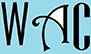## Algebra Question for Title 1 Schools: How Many Question?

1) If john can type a page in p minutes, how many pieces of paper can he type in 5 minutes?
distance = rate x time
rate = distance / time
1/p = x/5

you have to make “x” subject of formula by moving “5” to the other side. When you go to the other side, addition becomes subtraction, multiplication becomes division, and vice versa.
5/p = x (5 was a denominator at the other side, so it became multiplication)
John can do 5/p papers in 5 minutes